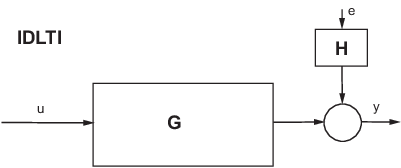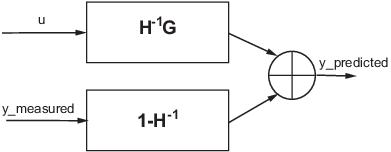Documentation

## About Identified Linear Models

### What are IDLTI Models?

System Identification Toolbox™ software uses objects to represent a variety of linear and nonlinear model structures. These linear model objects are collectively known as Identified Linear Time-Invariant (IDLTI) models.

IDLTI models contain two distinct dynamic components:

• Measured component — Describes the relationship between the measured inputs and the measured output (G)

• Noise component — Describes the relationship between the disturbances at the output and the measured output (H)

Models that only have the noise component `H` are called time-series or signal models. Typically, you create such models using time-series data that consist of one or more outputs `y(t)` with no corresponding input.

The total output is the sum of the contributions from the measured inputs and the disturbances: y = G u + H e, where u represents the measured inputs and e the disturbance. e(t) is modeled as zero-mean Gaussian white noise with variance Λ. The following figure illustrates an IDLTI model.When you simulate an IDLTI model, you study the effect of input u(t) (and possibly initial conditions) on the output y(t). The noise e(t) is not considered. However, with finite-horizon prediction of the output, both the measured and the noise components of the model contribute towards computation of the (predicted) response.

One-step ahead prediction model corresponding to a linear identified model (y = Gu+He)### Measured and Noise Component Parameterizations

The various linear model structures provide different ways of parameterizing the transfer functions `G` and `H`. When you construct an IDLTI model or estimate a model directly using input-output data, you can configure the structure of both G and H, as described in the following table:

Model TypeTransfer Functions G and H Configuration Method
State space model (`idss`)

Represents an identified state-space model structure, governed by the equations:

`$\begin{array}{l}\stackrel{˙}{x}=Ax+Bu+Ke\\ y=Cx+Du+e\end{array}$`

where the transfer function between the measured input u and output y is $G\left(s\right)=C{\left(sI-A\right)}^{-1}B+D$ and the noise transfer function is $H\left(s\right)=C{\left(sI-\text{​}A\right)}^{-1}K+I$.

Construction: Use `idss` to create a model, specifying values of state-space matrices A, B, C, D and K as input arguments (using `NaNs` to denote unknown entries).

Estimation: Use `ssest` or `n4sid`, specifying name-value pairs for various configurations, such as, canonical parameterization of the measured dynamics (`'Form'/'canonical'`), denoting absence of feedthrough by fixing D to zero (`'Feedthrough'/false`), and absence of noise dynamics by fixing K to zero (`'DisturbanceModel'/'none'`).

Polynomial model (`idpoly`)

Represents a polynomial model such as ARX, ARMAX and BJ. An ARMAX model, for example, uses the input-output equation Ay(t) = Bu(t)+Ce(t), so that the measured transfer function G is $G\left(s\right)={A}^{-1}B$, while the noise transfer function is $H\left(s\right)={A}^{-1}C$.

The ARMAX model is a special configuration of the general polynomial model whose governing equation is:

`$Ay\left(t\right)=\frac{B}{F}u\left(t\right)+\frac{C}{D}e\left(t\right)$`

The autoregressive component, A, is common between the measured and noise components. The polynomials B and F constitute the measured component while the polynomials C and D constitute the noise component.

Construction: Use `idpoly` to create a model using values of active polynomials as input arguments. For example, to create an Output-Error model which uses `G = B/F` as the measured component and has a trivial noise component (`H = 1`). enter:

`y = idpoly([],B,[],[],F)`

Estimation: Use the `armax`, `arx`, or `bj`, specifying the orders of the polynomials as input arguments. For example, `bj` requires you to specify the orders of the B, C, D, and F polynomials to construct a model with governing equation

`$y\left(t\right)=\frac{B}{F}u\left(t\right)+\frac{C}{D}e\left(t\right)$`

Transfer function model (`idtf`)

Represents an identified transfer function model, which has no dynamic elements to model noise behavior. This object uses the trivial noise model H(s) = I. The governing equation is

`$y\left(t\right)=\frac{num}{den}u\left(t\right)+e\left(t\right)$`

Construction: Use `idtf` to create a model, specifying values of the numerator and denominator coefficients as input arguments. The numerator and denominator vectors constitute the measured component `G = num(s)/den(s)`. The noise component is fixed to `H = 1`.

Estimation: Use `tfest`, specifying the number of poles and zeros of the measured component `G`.

Process model (`idproc`)

Represents a process model, which provides options to represent the noise dynamics as either first- or second-order ARMA process (that is, H(s)= C(s)/A(s), where C(s) and A(s) are monic polynomials of equal degree). The measured component, G(s), is represented by a transfer function expressed in pole-zero form.

For process (and grey-box) models, the noise component is often treated as an on-demand extension to an otherwise measured component-centric representation. For these models, you can add a noise component by using the `DisturbanceModel` estimation option. For example:

`model = procest(data,'P1D')`

estimates a model whose equation is:

`$y\left(s\right)={K}_{p}\frac{1}{\left({T}_{p1}s+1\right)}{e}^{-sTd}u\left(s\right)+e\left(s\right).$`

To add a second order noise component to the model, use:

```Options = procestOptions('DisturbanceModel','ARMA1'); model = procest(data,'P1D',Options); ```

This model has the equation:

`$y\left(s\right)={K}_{p}\frac{1}{\left({T}_{p1}s+1\right)}{e}^{-sTd}u\left(s\right)+\frac{1+{c}_{1}s}{1+{d}_{1}s}e\left(s\right)$`

where the coefficients c1 and d1 parameterize the noise component of the model. If you are constructing a process model using the `idproc` command, specify the structure of the measured component using the `Type` input argument and the noise component by using the `NoiseTF` name-value pair. For example,

```model = idproc('P1','Kp',1,'Tp1',1,'NoiseTF',... struct('num',[1 0.1],'den',[1 0.5]))```

creates the process model y(s) = 1/(s+1) u(s) + (s + 0.1)/(s + 0.5) e(s)

Sometimes, fixing coefficients or specifying bounds on the parameters are not sufficient. For example, you may have unrelated parameter dependencies in the model or parameters may be a function of a different set of parameters that you want to identify exclusively. For example, in a mass-spring-damper system, the `A` and `B` parameters both depend on the mass of the system. To achieve such parameterization of linear models, you can use grey-box modeling where you establish the link between the actual parameters and model coefficients by writing an ODE file. To learn more, see Grey-Box Model Estimation.

### Linear Model Estimation

You typically use estimation to create models in System Identification Toolbox. You execute one of the estimation commands, specifying as input arguments the measured data, along with other inputs necessary to define the structure of a model. To illustrate, the following example uses the state-space estimation command, `ssest`, to create a state space model. The first input argument `data` specifies the measured input-output data. The second input argument specifies the order of the model.

```sys = ssest(data,4) ```

The estimation function treats the noise variable e(t) as prediction error – the residual portion of the output that cannot be attributed to the measured inputs. All estimation algorithms work to minimize a weighted norm of e(t) over the span of available measurements. The weighting function is defined by the nature of the noise transfer function H and the focus of estimation, such as simulation or prediction error minimization.

#### Black Box (“Cold Start”) Estimation

In a black-box estimation, you only have to specify the order to configure the structure of the model.

```sys = estimator(data,orders) ```

where `estimator` is the name of an estimation command to use for the desired model type.

For example, you use `tfest` to estimate transfer function models, `arx` for ARX-structure polynomial models, and `procest` for process models.

The first argument, `data`, is time- or frequency domain data represented as an `iddata` or `idfrd` object. The second argument, `orders`, represents one or more numbers whose definitions depends upon the model type:

• For transfer functions, `orders` refers to the number of poles and zeros.

• For state-space models, `orders` refers to the number of states.

• For process models, `orders` denotes the structural elements of a process model, such as, the number of poles and presence of delay and integrator.

When working with the app, you specify the orders in the appropriate edit fields of corresponding model estimation dialogs.

#### Structured Estimations

In some situations, you want to configure the structure of the desired model more closely than what is achieved by simply specifying the orders. In such cases, you construct a template model and configure its properties. You then pass that template model as an input argument to the estimation commands in place of `orders`.

To illustrate, the following example assigns initial guess values to the numerator and the denominator polynomials of a transfer function model, imposes minimum and maximum bounds on their estimated values, and then passes the object to the estimator function.

```% Initial guess for numerator num = [1 2]; den = [1 2 1 1]; % Initial guess for the denominator sys = idtf(num,den); % Set min bound on den coefficients to 0.1 sys.Structure.Denominator.Minimum = [1 0.1 0.1 0.1]; sysEstimated = tfest(data,sys); ```

The estimation algorithm uses the provided initial guesses to kick-start the estimation and delivers a model that respects the specified bounds.

You can use such a model template to also configure auxiliary model properties such as input/output names and units. If the values of some of the model’s parameters are initially unknown, you can use `NaNs` for them in the template.

#### Estimation Options

There are many options associated with a model’s estimation algorithm that configure the estimation objective function, initial conditions and numerical search algorithm, among other things. For every estimation command, `estimator`, there is a corresponding option command named `estimator``Options`. To specify options for a particular estimator command, such as `tfest`, use the options command that corresponds to the estimation command, in this case, `tfestOptions`. The options command returns an options set that you then pass as an input argument to the corresponding estimation command.

For example, to estimate an Output-Error structure polynomial model, you use `oe`. To specify `simulation` as the focus and `lsqnonlin` as the search method, you use `oeOptions`:

```load iddata1 z1 Options = oeOptions('Focus','simulation','SearchMethod','lsqnonlin'); sys= oe(z1,[2 2 1],Options); ```

Information about the options used to create an estimated model is stored in the `OptionsUsed` field of the model’s `Report` property. For more information, see Estimation Report.

## Related Topics

##### SupportGet trial now